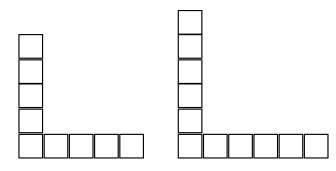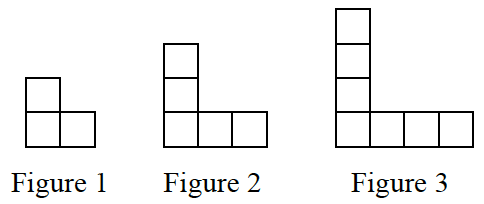### Home > CC4 > Chapter 1 > Lesson 1.1.1 > Problem1-8

1-8.

Examine the tile pattern at right. 1-8 HW eTool (CPM). Homework Help ✎

1. On your paper, sketch Figures 4 and 5.

How many tiles are in each leg of a figure if you don't count the tile in the corner? How does that compare to the figure number?2. How does the pattern grow? Explain how you know.

See the hints for part (a).

The pattern grows each time by two tiles, one on each arm of the figure. Explain how you know.

3. How many tiles will there be in Figure 0 (the figure before Figure 1)? Explain how you know.

Once you find the answer, remember to explain how you got to that number of tiles. Also, see part (b).Use the eTool below to examine and sketch the tile pattern.
Click the link to the right for a full version of the eTool: Int1 1-8 HW eTool